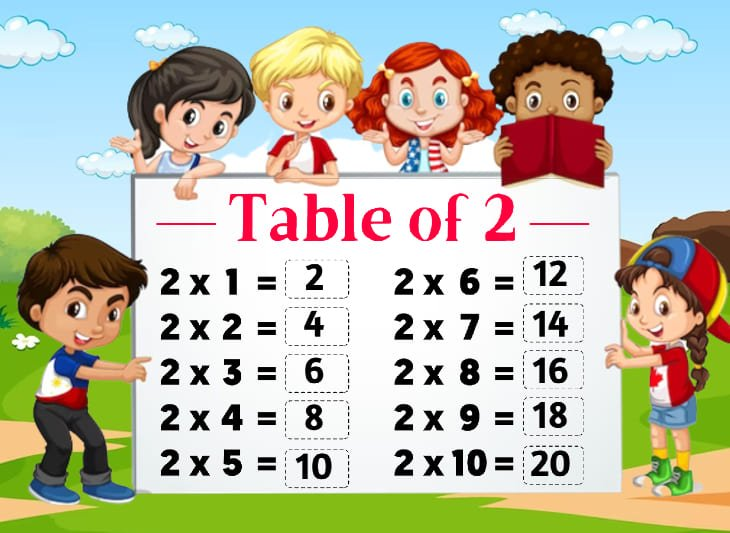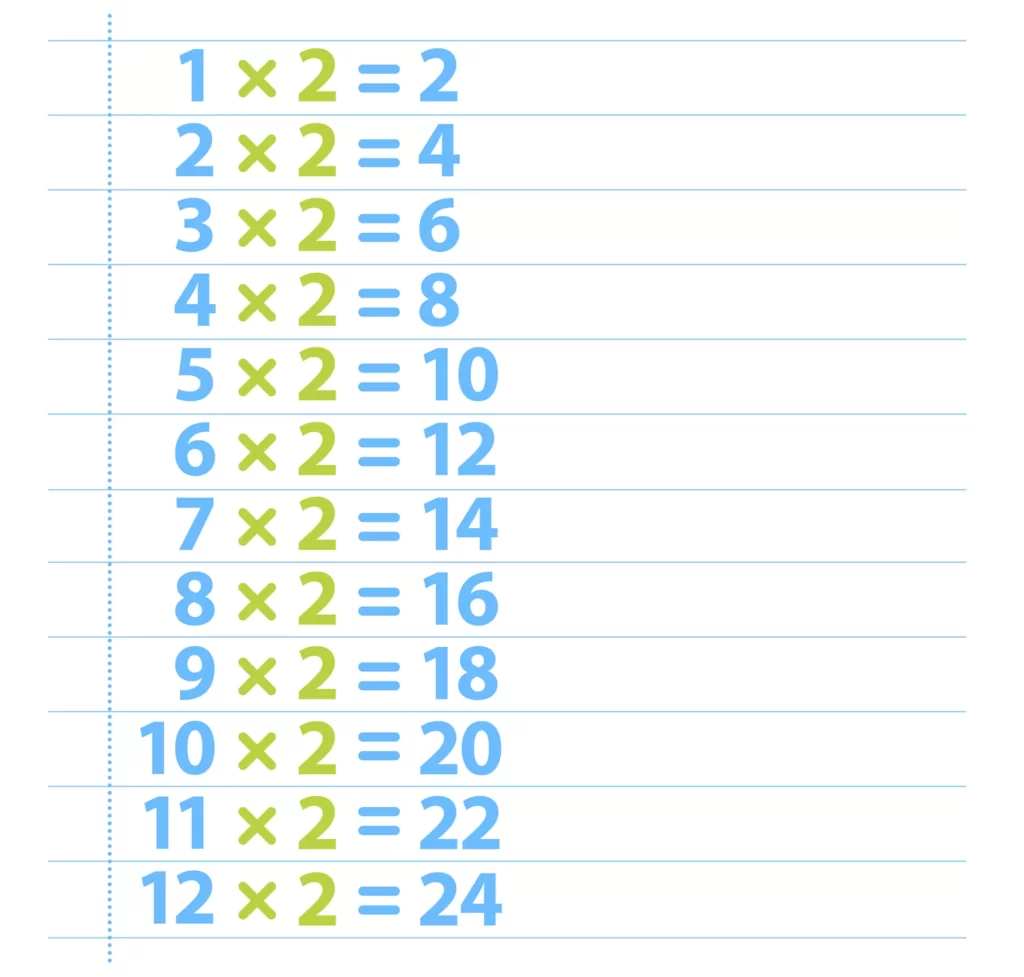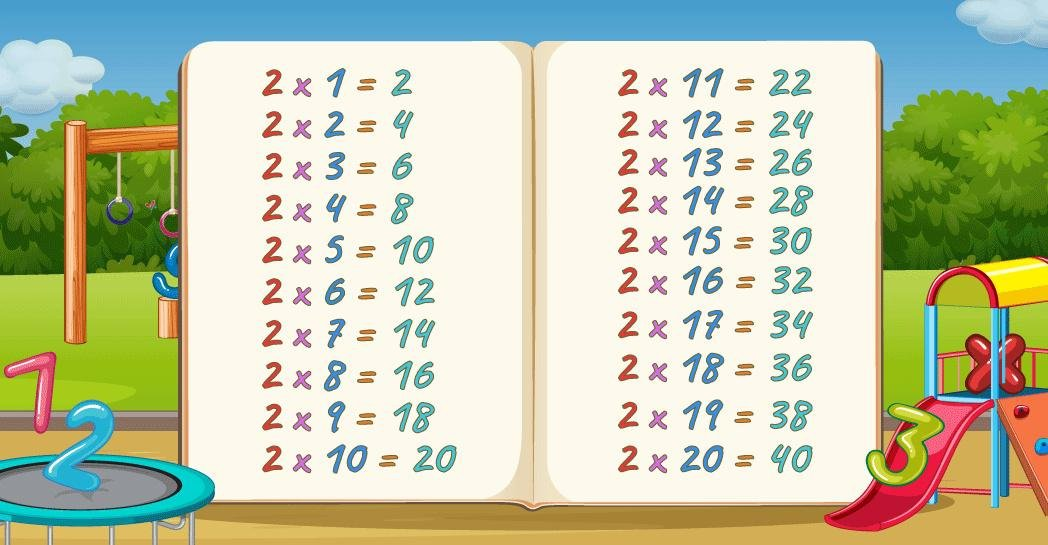# Times Table of 2, 2 ka table

## Times Table of 2, 2 ka table

Maths Multiplication Table of 2, Times Table of 2, 2 ka table, 2 ki Table Chart

2 ×‌ 1 = 2
2 ×‌ 2 = 4
2 × ‌3 = 6
2 × ‌4 = 8
2 × ‌5 = 10
2 × ‌6 = 12
2 × ‌7 = 14
2 × ‌8 = 16
2 × ‌9 = 18
2 × ‌10 = 20

## 2 ka table### Multiplication Tables of 2### Times Table of 2 chart